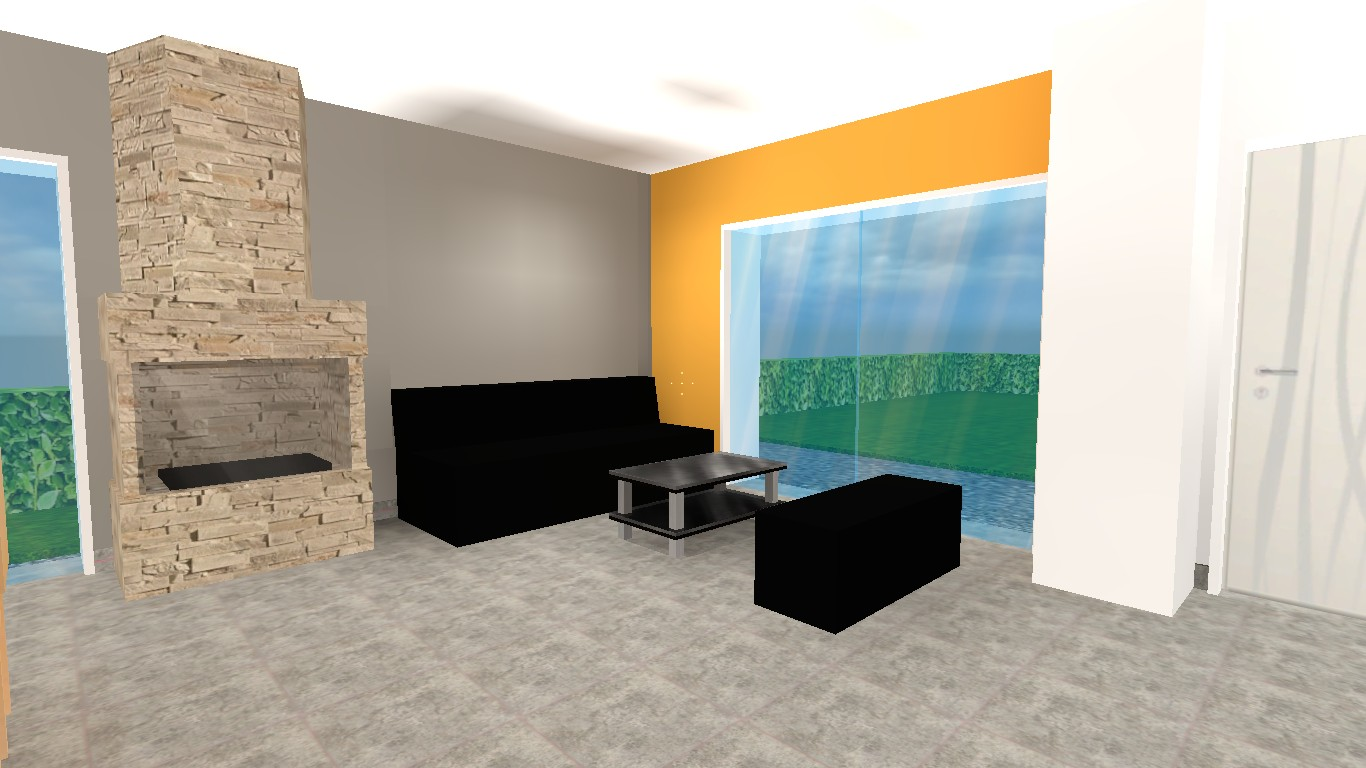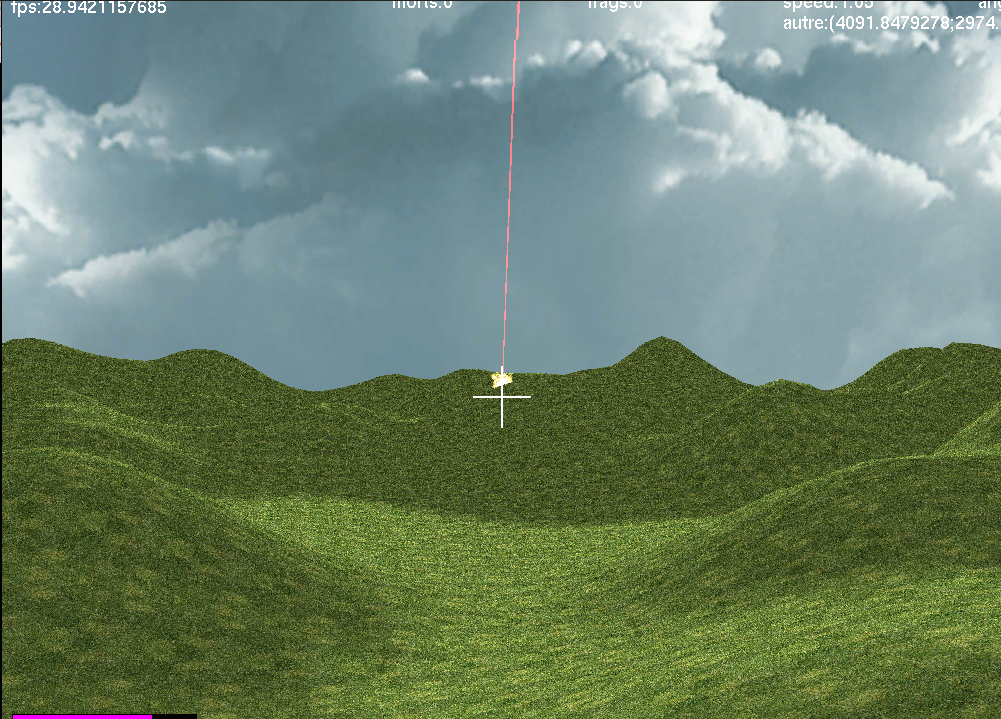Vincent Gripon's Homepage

Research and Teaching Blog

Graph Signal Processing

This webpage was coded by Chiraz Nafouki, during her internship at Télécom Bretagne.

Graph signal processing is a recent research domain that aim at generalizing classical signal processing. A graph is composed of a set of points, called nodes, such that some of them are connected through edges. If we label nodes from 1 to n, a graph is caracterized using an adjacency matrix. This matrix's cell (i,j) contains, if there is one, the connection weight connecting i and j, and 0 otherwise. A graph can be directed or not. An undirected graph has a symmetric adjacency matrix.
There are many application domains for graph signal processing. For instance the analysis of social networks, brain imaging, transport or power networks... Graphs are a generic model that can be applied everywhere.
Let us consider an example of a social network where nodes represent people and edges friendship relations between nodes:Example of a graph

Graph signal

A graph signal is a vector that associates with each node of the graph a real value. For example the signal can be the temperatures measured by sensors at different locations (nodes).

Representation in the spatial domain

There are many different ways to represent a signal in the spatial domain. Here we illustrate two of them: a 3D representation where signal values are depicted as bars with corresponding heights. The second example depicts signal values as color intensities. We use the latter in the following.2D representation

Spectral domain representation

Another matrix associated with a graph is the normalized Laplician, defined as: L = I - D-1/2 A D-1/2, where I is the identity matrix, D is the degree matrix and A the adjacency matrix. This operator can measure the smoothness of a signal on a graph using its quadratic form. As a matter of fact, eigenvalues of this operator are equivalent to "discrete frequencies" of signal decomposition using discrete Fourrier Transform. In the foregoing we denote by λi the i-th eigenvalue of the normalized Laplician with λ0≤λ1≤...≤λn-1 , where n is the order of the graph.

Graph signal diffusion

One can define a diffusion operator for signals supported by graphs. This matrix diffuses the signal so that asymptotically it almost surely converges to a nondegenerate value (neither infinity nor 0). Heat diffusion is one good example of this procedure.
The diffusion operator is W= D-1/2 A D-1/2 , where D is the degree matrix and A the adjacency matrix.
Given a graph and an initial signal on the graph, we propose to visualize the diffusion of the signal on the graph. For that, we multiply the signal iteratively by W.

Initial signal (nonnegative values)

Noeud 0 : Noeud 1 :

Noeud 2 : Noeud 3 :

Noeud 4 : Noeud 5 :

Noeud 6 : Noeud 7 :

Noeud 8 :

Visualizing diffusion

Each time you click on "Diffuse!" the signal is multiplied by W. Obtained signal is represented both in space and frequency.Home model using HL Source

Some views of a house construction project using Half-life source engine I realized.FPS in OCaml

A screenshot of a first-person-shooter I coded using OCaml. The network is also functionnal :)Personal Bépo layout

I use a modified bépo layout that I trained on my personal data.
You can find the console file here.
You can find the XKbmap here.
You can find the xmodmap file here.

Research locations map

Display Research locations on a larger map

Vincent Gripon's Homepage